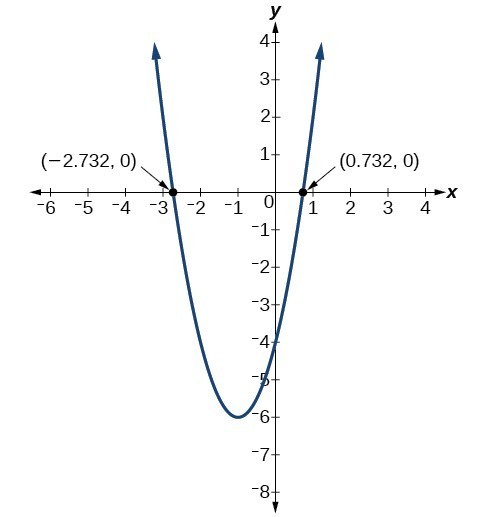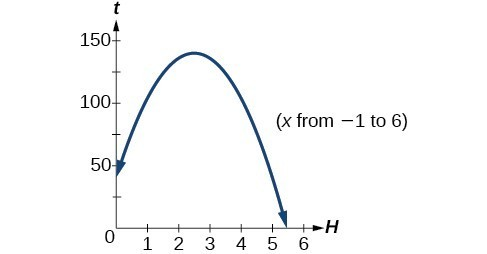## Solve problems involving a quadratic function’s minimum or maximum value

In Example 7, the quadratic was easily solved by factoring. However, there are many quadratics that cannot be factored. We can solve these quadratics by first rewriting them in standard form.

### How To: Given a quadratic function, find the x-intercepts by rewriting in standard form.

1. Substitute a and b into $h=-\frac{b}{2a}.\\$
2. Substitute xh into the general form of the quadratic function to find k.
3. Rewrite the quadratic in standard form using h and k.
4. Solve for when the output of the function will be zero to find the x-intercepts.

### Example 8: Finding the x-Intercepts of a Parabola

Find the x-intercepts of the quadratic function $f\left(x\right)=2{x}^{2}+4x - 4.\\$

### Solution

We begin by solving for when the output will be zero.

$0=2{x}^{2}+4x - 4\\$

Because the quadratic is not easily factorable in this case, we solve for the intercepts by first rewriting the quadratic in standard form.

$f\left(x\right)=a{\left(x-h\right)}^{2}+k\\$

We know that = 2. Then we solve for h and k.

$\begin{cases}h=-\frac{b}{2a}\hfill & \hfill & \hfill & k=f\left(-1\right)\hfill \\ \text{ }=-\frac{4}{2\left(2\right)}\hfill & \hfill & \hfill & \text{ }=2{\left(-1\right)}^{2}+4\left(-1\right)-4\hfill \\ \text{ }=-1\hfill & \hfill & \hfill & \text{ }=-6\hfill \end{cases}\\$

So now we can rewrite in standard form.

$f\left(x\right)=2{\left(x+1\right)}^{2}-6\\$

We can now solve for when the output will be zero.

$\begin{cases}0=2{\left(x+1\right)}^{2}-6\hfill \\ 6=2{\left(x+1\right)}^{2}\hfill \\ 3={\left(x+1\right)}^{2}\hfill \\ x+1=\pm \sqrt{3}\hfill \\ x=-1\pm \sqrt{3}\hfill \end{cases}\\$

The graph has x-intercepts at $\left(-1-\sqrt{3},0\right)\\$ and $\left(-1+\sqrt{3},0\right).\\$

### Analysis of the SolutionFigure 15

We can check our work by graphing the given function on a graphing utility and observing the x-intercepts.

### Try It 4

In Try It 2, we found the standard and general form for the function $g\left(x\right)=13+{x}^{2}-6x.\\$ Now find the y– and x-intercepts (if any).

Solution

Solve ${x}^{2}+x+2=0.\\$

### Solution

Let’s begin by writing the quadratic formula: $x=\frac{-b\pm \sqrt{{b}^{2}-4ac}}{2a}.\\$

When applying the quadratic formula, we identify the coefficients ab, and c. For the equation ${x}^{2}+x+2=0,\\$ we have a = 1, b = 1, and c = 2. Substituting these values into the formula we have:

$\begin{cases}x=\frac{-b\pm \sqrt{{b}^{2}-4ac}}{2a}\hfill \\ \text{ }=\frac{-1\pm \sqrt{{1}^{2}-4\cdot 1\cdot \left(2\right)}}{2\cdot 1}\hfill \\ \text{ }=\frac{-1\pm \sqrt{1 - 8}}{2}\hfill \\ \text{ }=\frac{-1\pm \sqrt{-7}}{2}\hfill \\ \text{ }=\frac{-1\pm i\sqrt{7}}{2}\hfill \end{cases}\\$

The solutions to the equation are $x=\frac{-1+i\sqrt{7}}{2}\\$ and $x=\frac{-1-i\sqrt{7}}{2}\\$ or $x=\frac{-1}{2}+\frac{i\sqrt{7}}{2}\\$ and $x=\frac{-1}{2}-\frac{i\sqrt{7}}{2}.\\$

### Example 10: Applying the Vertex and x-Intercepts of a Parabola

A ball is thrown upward from the top of a 40 foot high building at a speed of 80 feet per second. The ball’s height above ground can be modeled by the equation $H\left(t\right)=-16{t}^{2}+80t+40.\\$

a. When does the ball reach the maximum height?

b. What is the maximum height of the ball?

c. When does the ball hit the ground?

### Solution

a. The ball reaches the maximum height at the vertex of the parabola.

$\begin{cases} h=-\frac{80}{2\left(-16\right)} \text{ }=\frac{80}{32}\hfill \\ \text{ }=\frac{5}{2}\hfill \\ \text{ }=2.5\hfill \end{cases}\\$

The ball reaches a maximum height after 2.5 seconds.

b. To find the maximum height, find the coordinate of the vertex of the parabola.

$\begin{cases}k=H\left(-\frac{b}{2a}\right)\hfill \\ \text{ }=H\left(2.5\right)\hfill \\ \text{ }=-16{\left(2.5\right)}^{2}+80\left(2.5\right)+40\hfill \\ \text{ }=140\hfill \end{cases}\\$

The ball reaches a maximum height of 140 feet.

c. To find when the ball hits the ground, we need to determine when the height is zero, $H\left(t\right)=0.\\$

$\begin{cases} t=\frac{-80\pm \sqrt{{80}^{2}-4\left(-16\right)\left(40\right)}}{2\left(-16\right)}\hfill \\ \text{ }=\frac{-80\pm \sqrt{8960}}{-32}\hfill \end{cases}\\$

Because the square root does not simplify nicely, we can use a calculator to approximate the values of the solutions.

$\begin{cases}t=\frac{-80-\sqrt{8960}}{-32}\approx 5.458\hfill & \text{or}\hfill & t=\frac{-80+\sqrt{8960}}{-32}\approx -0.458\hfill \end{cases}$

The second answer is outside the reasonable domain of our model, so we conclude the ball will hit the ground after about 5.458 seconds.Figure 16

### Try It 5

A rock is thrown upward from the top of a 112-foot high cliff overlooking the ocean at a speed of 96 feet per second. The rock’s height above ocean can be modeled by the equation $H\left(t\right)=-16{t}^{2}+96t+112.\\$

a. When does the rock reach the maximum height?

b. What is the maximum height of the rock?

c. When does the rock hit the ocean?

Solution